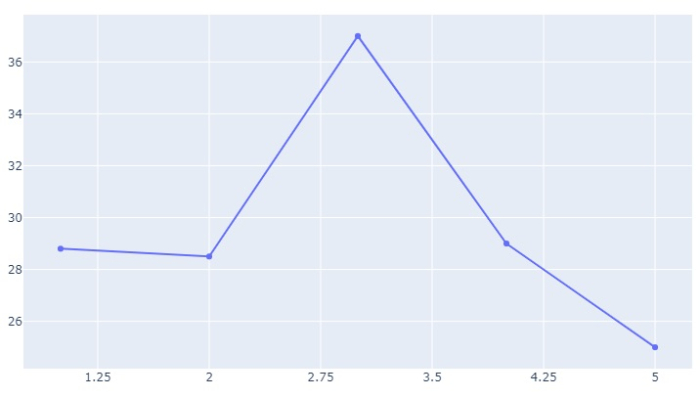# How to show all the X-axis tick values in Python Plotly?

Plotly is an open-source Python library for creating charts. In this tutorial, we will show how you can use the features of Plotly to display specific axis tick values. Let us understand how to show all the X-axis tick values,

• Use plotly.graph_objects to generate figures. It contains a lot of methods to customize chart and render a chart into HTML format.

• Update_layout() method has X-axis parameter and the value must be assigned as tickmode='linear'.

Follow the steps given below to show all the X-axis tick values.

Step 1

Import plotly.graphs_objs module and alias as go

import plotly.graphs_objs as go

Step 2

Create a scatter plot using go.Figure() method as follows,

# create a scatter plot
fig = go.Figure(go.Scatter(
x = [1, 2, 3, 4, 5],
y = [28.8, 28.5, 37, 29, 25]
))

Step 3

Create the update_layout() method to set the X-axis dict values.

# update layout for xaxis tickmode as linear
fig.update_layout(
xaxis = dict(
tickmode = 'linear',
tick0 = 0.5,
dtick = 0.75
)
)

## Example

The complete code to display all the X-axis tick value is as follows −

import plotly.graph_objects as go

# create a scatter plot
fig = go.Figure(go.Scatter(
x = [1, 2, 3, 4, 5],
y = [28.8, 28.5, 37, 29, 25]
))

# update layout for xaxis tickmode as linear
fig.update_layout(
xaxis = dict(
tickmode = 'linear',
tick0 = 0.5,
dtick = 0.75
)
)

## Output

It will show the following output on the browser −